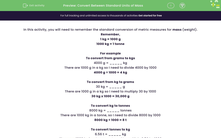# Convert Between Standard Units of Mass

In this worksheet, students will be asked to convert between standard units of mass using metric measures and solve problems using this knowledge.Key stage:  KS 2

Curriculum topic:   Measurement

Curriculum subtopic:   Convert Between Standard Units

Difficulty level:#### Worksheet Overview

In this activity, you will need to remember the standard conversion of metric measures for mass (weight).

Remember,

1 kg = 1000 g

1000 kg = 1 tonne

For example

To convert from grams to kgs

4000 g = _____ kg

There are 1000 g in a kg so I need to divide 4000 by 1000

4000 g ÷ 1000 = 4 kg

To convert from kg to grams

30 kg = _____ g

There are 1000 g in a kg so I need to multiply 30 by 1000

30 kg x 1000 = 30,000 g

To convert kg to tonnes

8000 kg = _____ tonnes

There are 1000 kg in a tonne, so I need to divide 8000 by 1000

8000 kg ÷ 1000 = 8 t

To convert tonnes to kg

6.56 t = _____ kg

There are 1000 kg in a tonne, so I need to multiply 6.56 by 1000

6.56 t x 1000 = 6560 kg

### What is EdPlace?

We're your National Curriculum aligned online education content provider helping each child succeed in English, maths and science from year 1 to GCSE. With an EdPlace account you’ll be able to track and measure progress, helping each child achieve their best. We build confidence and attainment by personalising each child’s learning at a level that suits them.

Get started